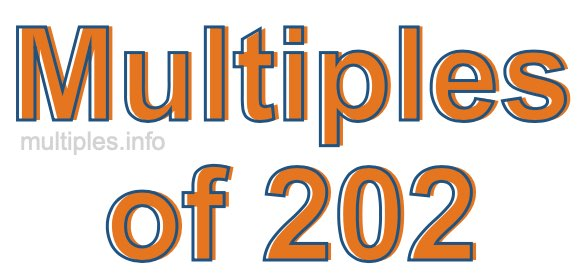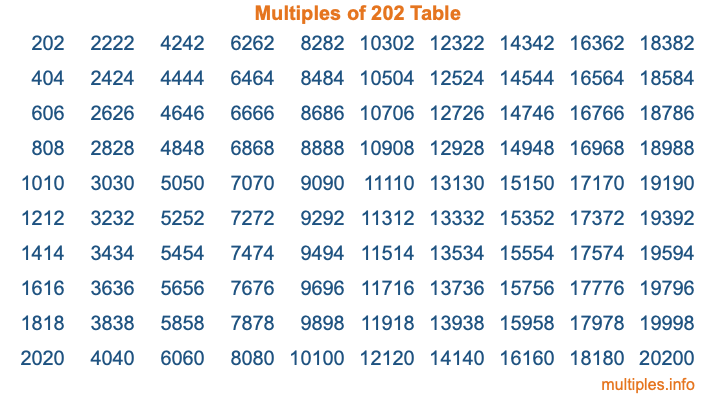Multiples of 202Welcome to the Multiples of 202 page. Here we will first teach you everything you will ever need to know about the multiples of 202, and then give you a study guide summary of everything we taught you to make sure you remember it all. Use this page to look up facts and learn information about the multiples of 202. This page will make you a multiples of two hundred two expert!

Definition of Multiples of 202
Multiples of 202 are all the numbers that when divided by 202 equal an integer. Each of the multiples of 202 are called a multiple. A multiple of 202 is created by multiplying 202 by an integer.

Therefore, to create a list of multiples of 202, you start with 1 multiplied by 202, then 2 multiplied by 202, then 3 multiplied by 202, and so on for as long as you want. Thus, the list of the first five multiples of 202 is 202, 404, 606, 808, and 1010. To see a larger list of multiples of 202, see the printable image of Multiples of 202 further down on this page. We also have a category where you can choose any nth multiple of 202.

Multiples of 202 Checker
The Multiples of 202 Checker below checks to see if any number of your choice is a multiple of 202. In other words, it checks to see if there is any number (integer) that when multiplied by 202 will equal your number. To do that, we divide your number by 202. If the the quotient is an integer, then your number is a multiple of 202.

Is  a multiple of 202?

Least Common Multiple of 202 and ...
A Least Common Multiple (LCM) is the lowest multiple that two or more numbers have in common. This is also called the smallest common multiple or lowest common multiple and is useful to know when you are adding our subtracting fractions. Enter one or more numbers below (202 is already entered) to find the LCM.

Check out our LCM Calculator if you need more details about the Least Common Multiple or if you need the LCM for different numbers for adding and subtraction fractions.

nth Multiple of 202
As we stated above, 202 is the first multiple of 202, 404 is the second multiple of 202, 606 is the third multiple of 202, and so on. Enter a number below to find the nth multiple of 202.

th multiple of 202

Multiples of 202 vs Factors of 202
202 is a multiple of 202 and a factor of 202, but that is where the similarities end. All postive multiples of 202 are 202 or greater than 202. All positive factors of 202 are 202 or less than 202.

Below is the beginning list of multiples of 202 and the factors of 202 so you can compare:

Multiples of 202: 202, 404, 606, 808, 1010, etc.

Factors of 202: 1, 2, 101, 202

As you can see, the multiples of 202 are all the numbers that you can divide by 202 to get a whole number. The factors of 202, on the other hand, are all the whole numbers that you can multiply by another whole number to get 202.

It's also interesting to note that if a number (x) is a factor of 202, then 202 will also be a multiple of that number (x).

Multiples of 202 vs Divisors of 202
The divisors of 202 are all the integers that 202 can be divided by evenly. Below is a list of the divisors of 202.

Divisors of 202: 1, 2, 101, 202

The interesting thing to note here is that if you take any multiple of 202 and divide it by a divisor of 202, you will see that the quotient is an integer.

Multiples of 202 Table
Below is an image of the first 100 multiples of 202 in a table. The table is in chronological order, column by column. The first column has the first ten multiples of 202, the second column has the next ten multiples of 202, and so on.The Multiples of 202 Table is also referred to as the 202 Times Table or Times Table of 202. You are welcome to print out our table for your studies.

Negative Multiples of 202
Although not often discussed or needed in math, it is worth mentioning that you can make a list of negative multiples of 202 by multiplying 202 by -1, then by -2, then by -3, and so on, to get the following list of negative multiples of 202:

-202, -404, -606, -808, -1010, etc.

Multiples of 202 Summary
Below is a summary of important Multiples of 202 facts that we have discussed on this page. To retain the knowledge on this page, we recommend that you read through the summary and explain to yourself or a study partner why they hold true.

There are an infinite number of multiples of 202.

A multiple of 202 divided by 202 will equal a whole number.

202 divided by a factor of 202 equals a divisor of 202.

The nth multiple of 202 is n times 202.

The largest factor of 202 is equal to the first positive multiple of 202.

202 is a multiple of every factor of 202.

202 is a multiple of 202.

A multiple of 202 divided by a divisor of 202 equals an integer.

202 divided by a divisor of 202 equals a factor of 202.

Any integer times 202 will equal a multiple of 202.

Multiples of a Number
Here you can get the multiples of another number, all with the same attention to detail as we did for multiples of 202 on this page.

Multiples of
Multiples of 203
Did you find our page about multiples of two hundred two educational? Do you want more knowledge? Check out the multiples of the next number on our list!